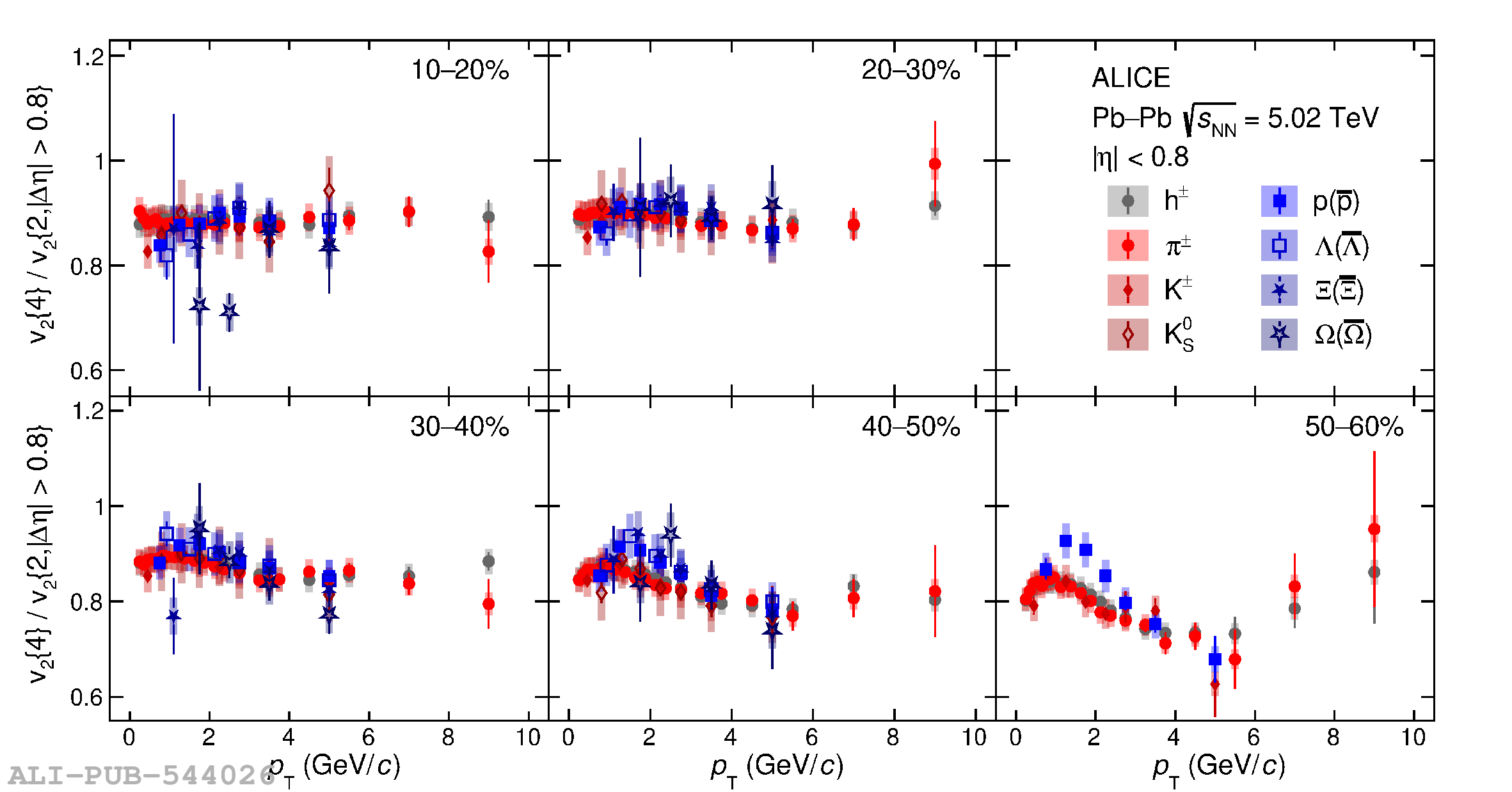# Figure 8

 The ratio $v_2\{4\}/v_2\{2,|\Delta \eta| > 0.8\}$ as a function of \pt{} for different particle species and centralities in Pb--Pb collisions at $\sqrt{s_{\mathrm{NN}}} = 5.02$ TeV. The vertical error bars and the filled boxes represent statistical and systematic uncertainties, respectively.